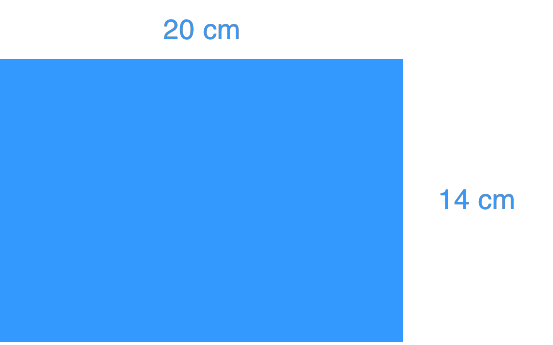# Squares and square roots

### Squares and square roots

This lesson focuses on finding the square of a whole number and the square root of a perfect square. In order to do this, we will first learn how to do prime factorization, a method to find the prime factors of a number.

#### Lessons

• Introduction
a)
• What is a square root?
• How to find side lengths of squares using square roots?
• How to estimate non-perfect squares?

b)
What is a perfect square?

• 1.
Find the prime factorization. Then, identify the perfect squares.
a)
53

b)
71

c)
196

• 2.
Find the area of the square that has a side length of:
a)
15 cm

b)
21 cm

• 3.
Calculate.
a)
$\sqrt {16}$

b)
$\sqrt {49}$

c)
$\sqrt {2025}$

• 4.a)
Find the area of the rectangle.

b)
What is the side length of a square that has the same area as the rectangle above?